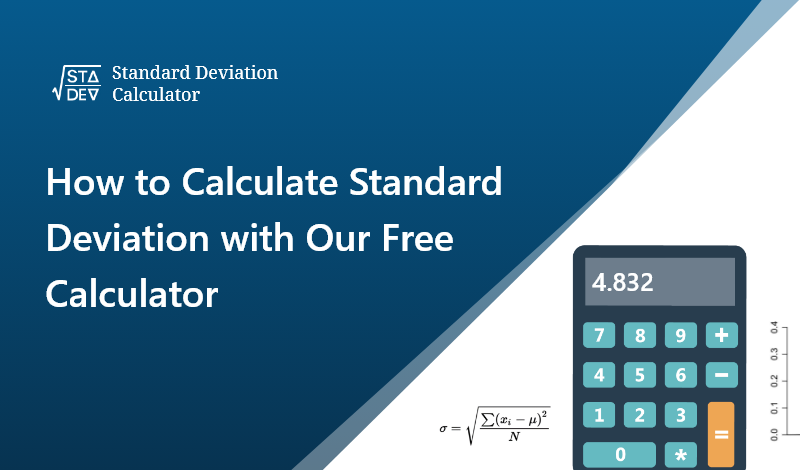May 29, 2023
How to Calculate Standard Deviation with Our Free Calculator?The standard deviation is an extremely important statistical measure that tells us how much variation or dispersion exists from the average (mean) in a set of data. In simpler terms, it gives us an idea of how far individual measurements are likely to be from the mean.

Understanding the standard deviation can be critical in many fields, from finance to psychology, medicine to engineering. Despite its importance, calculating standard deviation by hand can be a complex and tedious process. But what if there were a simpler way?

## Introducing our free online tool: The Standard Deviation Calculator

This tool is designed to make your standard deviation calculations as effortless as possible. Here's how to use it:

Step 1:

Gather Your Data The first step in calculating standard deviation is to gather your data. This data can be anything from test scores, to financial returns, or even measurements of physical objects. Be sure your data is accurate as this will ensure the most accurate calculation.

Step 2:

Enter Your Data Once you're on the page, you'll see a large, easy-to-use interface. Look for a box labeled 'Enter your data'. You can input your data here, making sure to separate each data point with a comma. For instance, if your data set is 1, 2, 3, 4, and 5, you'd enter it as 1,2,3,4,5.

Step 3:

Calculate After you've entered your data, click on the 'Calculate' button. The tool will automatically process your data and display the result. The result is the standard deviation of your dataset.

The website not only provides you with the standard deviation but also offers additional information. It provides the variance, mean, sum, and count of your data points. This gives a more complete statistical understanding of your data.

Step 4:

Interpret the Result Once you have the standard deviation, you can interpret the results. A lower standard deviation means that the data points are close to the mean, indicating less variability or dispersion in the data set. Conversely, a high standard deviation indicates that the data points are spread out over a wider range.

Our free Standard Deviation Calculator makes it simple and quick to calculate the standard deviation of any dataset. No longer do you have to go through the tedious process of calculating it by hand? All you need is your data, and our tool does the rest. Understanding your data has never been easier!

It's time to take the guesswork out of your data analysis and let our free Standard Deviation Calculator do the work for you. Give it a try today!